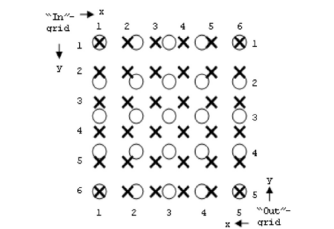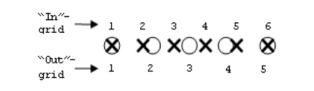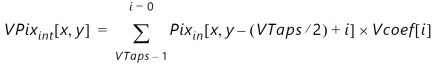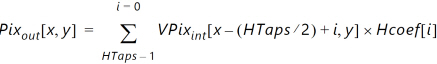# Polyphase Scaling - 2.4 English

## Video Processing Subsystem Product Guide (PG231)

Document ID
PG231
Release Date
2022-10-19
Version
2.4 English

For scaling, the input and output sampling grids are assumed to be different. To express a discrete output pixel in terms of input pixels, it is necessary to know or estimate the location of the output pixel relative to the closest input pixels when superimposing the output sampling grid upon the input sampling grid for the equivalent 2-D space. With this knowledge, the algorithm approximates the output pixel value by using a filter with coefficients weighted accordingly. Filter taps are consecutive data-points drawn from the input image.

As an example, This Figure shows a desired 5x5 output grid ("O") superimposed upon an original 6x6 input grid ("X"), occupying common space. In this case, estimating for output position (x, y) = (1, 1), shows the input and output pixels to be co-located. You can weigh the coefficients to reflect no bias in either direction, and can even select a unity coefficient set. Output location (2, 2) is offset from the input grid in both vertical and horizontal dimensions. Coefficients can be chosen to reflect this, most likely showing some bias towards input pixel (2, 2), etc. Filter characteristics can be built into the filter coefficients by appropriately applying anti-aliasing low-pass filters.

 X-Ref Target - Figure 3-4The space between two consecutive input pixels in each dimension is conceptually partitioned into a number of bins or phases. The location of any arbitrary output pixel always falls into one of these bins, thus defining the phase of coefficients used. The filter architecture should be able to accept any of the different phases of coefficients, changing phase on a sample-by-sample basis.

A single dimension is shown in This Figure . As illustrated in this figure, the five output pixels shown from left to right could have the phases 0, 1, 2, 3, 0.

 X-Ref Target - Figure 3-5The examples in This Figure and This Figure show a conversion where the ratio Xin / Xout = Yin / Yout = 5/4. This ratio is known as the scaling factor, or SF. The horizontal and vertical Scaling Factors can be different. A typical example is drawn from the broadcast industry, where some footage can be shot using 720p (1280 x 720), but the cable operator needs to deliver it as per the broadcast standard 1080p (1920 x 1080). The SF becomes 2/3 in both H and V dimensions.

Typically, when Xin > Xout , this conversion is known as horizontal down-scaling (SF > 1). When Xin < Xout , it is known as horizontal up-scaling (SF < 1).

The set of coefficients constitute filter banks in a polyphase filter whose frequency response is determined by the amount of scaling applied to the input samples. The phases of the filter represent subfilters for the set of samples in the final scaled result.

The number of coefficients and their values are dependent upon the required low-pass, anti-alias response of the scaling filter; for example, smaller scaling ratios require lower passbands and more coefficients. Filter design programs based on the Lanczos algorithm are suitable for coefficient generation. Moreover, MATLAB ® product fdatool/fvtool can be used to provide a wider filter design toolset.

A general guideline is to use 4 taps per number of scaling ratio for scaling down to get good quality. The following are some recommendations for how many taps to use:

 Upscale 6 taps Down scale to 1.5 6 taps Down scale > 1.5 <= 2.5 8 taps Down scale > 2.5 <= 3.5 10 taps Down scale > 3.5 12 taps

A direct implementation of Equation 1 suggests that a filter with VTaps x HTaps multiply operations per output are required. However, the Xilinx ® Video Scaler supports only separable filters, which completes an approximation of the 2-D operation using two 1-D stages in sequence - a vertical filter (V-filter) stage and a horizontal filter (H-filter) stage. The intermediate results of the first stage are fed sequentially to the second stage.

The vertical filter stage filters only in the vertical domain, for each incrementing horizontal raster scan position x , creating an intermediate result described as VPix ( This Equation ).

Equation 3-2The output result of the vertical component of the scaler filter is input into the horizontal filter with the appropriate rounding applied. The separation means this can be reduced to the shown VTaps and HTaps multiply operations, saving FPGA resources ( This Equation ).

Equation 3-3Notice that the differences between the Bilinear, Bicubic, and Polyphase architectures are not only marked by a difference in coefficients but with the implementation of optimized architectures for Bilinear and Bicubic scaling.